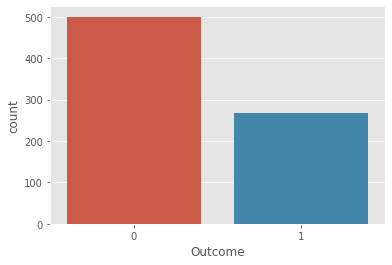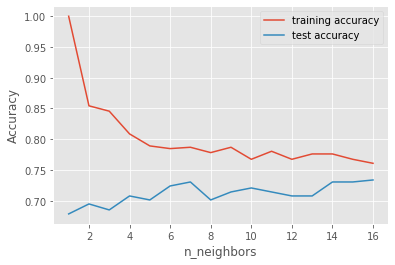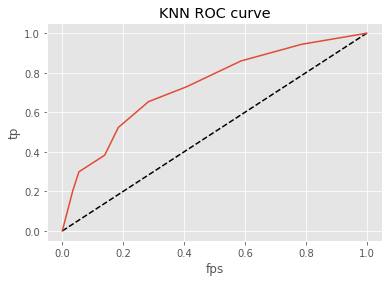In :
#kNN Classifier implementation – Load the data

import numpy as np
import pandas as pd
import matplotlib
matplotlib.use
import matplotlib.pyplot as plt
plt.style.use('ggplot')
print("Started")

Started


The diabetes data set was originated from UCI Machine Learning Repository and can be downloaded from https://github.com/susanli2016/Machine-Learning-with-Python/blob/master/diabetes.csv

In :
#load the dataset

#print the first 6 rows from dataframe

Out:
Pregnancies Glucose BloodPressure SkinThickness Insulin BMI DiabetesPedigreeFunction Age Outcome
0 6 148 72 35 0 33.6 0.627 50 1
1 1 85 66 29 0 26.6 0.351 31 0
2 8 183 64 0 0 23.3 0.672 32 1
3 1 89 66 23 94 28.1 0.167 21 0
4 0 137 40 35 168 43.1 2.288 33 1
5 5 116 74 0 0 25.6 0.201 30 0
In :
data.dtypes

Out:
Pregnancies                   int64
Glucose                       int64
BloodPressure                 int64
SkinThickness                 int64
Insulin                       int64
BMI                         float64
DiabetesPedigreeFunction    float64
Age                           int64
Outcome                       int64
dtype: object

The diabetes data set consists of 768 data points, with 9 features each:

In :
#Shape of dataframe
data.shape

Out:
(768, 9)
In :
X= data.drop('Outcome', axis=1).values
y = data['Outcome'].values

In :
X.shape

Out:
(768, 8)
In :
y.shape

Out:
(768,)

“Outcome” is the feature we are going to predict, 0 means No diabetes, 1 means diabetes. Of these 768 data points, 500 are labeled as 0 and 268 as 1:

In :
print(data.groupby('Outcome').size())

Outcome
0    500
1    268
dtype: int64

In :
import seaborn as sns
sns.countplot(data['Outcome'],label="Count")

Out:
<matplotlib.axes._subplots.AxesSubplot at 0x1d1f0701cc8>### kNN Classifier implementation – Split the dataset

In :
from sklearn.model_selection import train_test_split

X_train,X_test,y_train,y_test=train_test_split(X,y,test_size=0.4,random_state=42,stratify=y)
X_train.shape

Out:
(460, 8)

# kNN Classifier implementation – Build/Train model for broad range of k

In :
from sklearn.neighbors import KNeighborsClassifier

In :
training_accuracy = []
test_accuracy = []
# try n_neighbors from 1 to 10
neighbors_settings = range(1, 17)
for n_neighbors in neighbors_settings:
# build the model
knn = KNeighborsClassifier(n_neighbors=n_neighbors)
knn.fit(X_train, y_train)
# record training set accuracy
training_accuracy.append(knn.score(X_train, y_train))
# record test set accuracy
test_accuracy.append(knn.score(X_test, y_test))

#kNN Classifier implementation – Plot the accuracy

plt.plot(neighbors_settings, training_accuracy, label="training accuracy")
plt.plot(neighbors_settings, test_accuracy, label="test accuracy")
plt.ylabel("Accuracy")
plt.xlabel("n_neighbors")
plt.legend()
plt.savefig('knn_compare_model')The above plot shows the training and test set accuracy on the y-axis against the setting of n_neighbors on the x-axis. Considering if we choose one single nearest neighbor, the prediction on the training set is perfect. But when more neighbors are considered, the training accuracy drops, indicating that using the single nearest neighbor leads to a model that is too complex. The best performance is somewhere around 9 neighbors. The plot suggests that we should choose n_neighbors=9. Here we are:

### kNN Classifier implementation – Fit the model for observed K

In :
knn = KNeighborsClassifier(n_neighbors=9)
knn.fit(X_train, y_train)

Out:
KNeighborsClassifier(algorithm='auto', leaf_size=30, metric='minkowski',
metric_params=None, n_jobs=None, n_neighbors=9, p=2,
weights='uniform')
In :
knn.score(X_test,y_test)

Out:
0.7142857142857143
In :
knn.score(X_train,y_train)

Out:
0.7869565217391304

### kNN Classifier implementation – Measure the performance of classifier

In :
y_pred = knn.predict(X_test)

In :
pd.crosstab(y_test,y_pred,rownames=['True'],colnames=['Predicted'],margins=True)

Out:
Predicted 0 1 All
True
0 164 37 201
1 51 56 107
All 215 93 308
In :
from sklearn.metrics import classification_report
print(classification_report(y_test,y_pred))

              precision    recall  f1-score   support

0       0.76      0.82      0.79       201
1       0.60      0.52      0.56       107

accuracy                           0.71       308
macro avg       0.68      0.67      0.67       308
weighted avg       0.71      0.71      0.71       308



### kNN Classifier implementation – Plot ROC Curve

In :
y_pred_prob = knn.predict_proba(X_test)[:,1]

In :
from sklearn.metrics import roc_curve

In :
fps,tp,threshold = roc_curve(y_test,y_pred_prob)

In :
plt.plot([0,1],[0,1],'k--')
plt.plot(fps,tp,label='KNN')
plt.xlabel('fps')
plt.ylabel('tp')
plt.title('KNN ROC curve')
plt.show()

from sklearn.metrics import roc_auc_score
roc_auc_score(y_test,y_pred_prob)Out:
0.7320407309248153

### kNN Classifier implementation – Hyperparameter Tuning & Cross Validation

In :
from sklearn.model_selection import GridSearchCV
param_grid = {'n_neighbors':np.arange(1,50)}
knn= KNeighborsClassifier()
knn_cv = GridSearchCV(knn,param_grid,cv=5)
knn_cv.fit(X,y)

Out:
GridSearchCV(cv=5, error_score='raise-deprecating',
estimator=KNeighborsClassifier(algorithm='auto', leaf_size=30,
metric='minkowski',
metric_params=None, n_jobs=None,
n_neighbors=5, p=2,
weights='uniform'),
iid='warn', n_jobs=None,
param_grid={'n_neighbors': array([ 1,  2,  3,  4,  5,  6,  7,  8,  9, 10, 11, 12, 13, 14, 15, 16, 17,
18, 19, 20, 21, 22, 23, 24, 25, 26, 27, 28, 29, 30, 31, 32, 33, 34,
35, 36, 37, 38, 39, 40, 41, 42, 43, 44, 45, 46, 47, 48, 49])},
pre_dispatch='2*n_jobs', refit=True, return_train_score=False,
scoring=None, verbose=0)
In :
knn_cv.best_score_

Out:
0.7578125
In :
knn_cv.best_params_

Out:
{'n_neighbors': 14}
In :
knn = KNeighborsClassifier(n_neighbors=14)
knn.fit(X_train, y_train)
print('Accuracy of K-NN classifier on training set: {:.2f}'.format(knn.score(X_train, y_train)))
print('Accuracy of K-NN classifier on test set: {:.2f}'.format(knn.score(X_test, y_test)))

Accuracy of K-NN classifier on training set: 0.78
Accuracy of K-NN classifier on test set: 0.73

In :
 ! jupyter nbconvert --to html Untitled.ipynb

[NbConvertApp] Converting notebook Untitled.ipynb to html
[NbConvertApp] Writing 340423 bytes to Untitled.html

In [ ]: